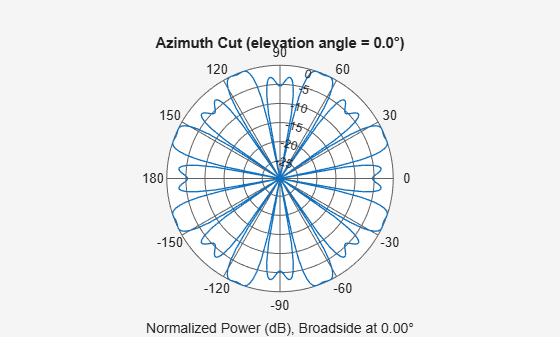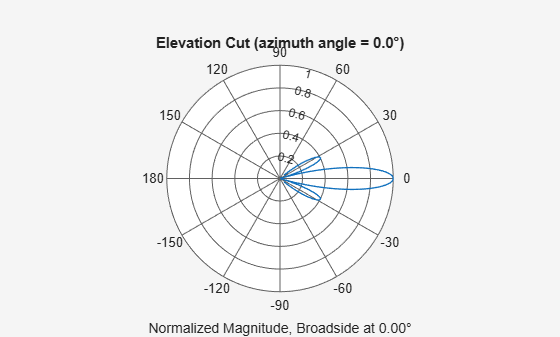# pattern

System object: phased.ConformalArray
Package: phased

Plot conformal array pattern

## Syntax

```pattern(sArray,FREQ) pattern(sArray,FREQ,AZ) pattern(sArray,FREQ,AZ,EL) pattern(___,Name,Value) [PAT,AZ_ANG,EL_ANG] = pattern(___) ```

## Description

`pattern(sArray,FREQ)` plots the 3-D array directivity pattern (in dBi) for the array specified in `sArray`. The operating frequency is specified in `FREQ`.

The integration used when computing array directivity has a minimum sampling grid of 0.1 degrees. If an array pattern has a beamwidth smaller than this, the directivity value will be inaccurate.

`pattern(sArray,FREQ,AZ)` plots the array directivity pattern at the specified azimuth angle.

`pattern(sArray,FREQ,AZ,EL)` plots the array directivity pattern at specified azimuth and elevation angles.

`pattern(___,Name,Value)` plots the array pattern with additional options specified by one or more `Name,Value` pair arguments.

`[PAT,AZ_ANG,EL_ANG] = pattern(___)` returns the array pattern in `PAT`. The `AZ_ANG` output contains the coordinate values corresponding to the rows of `PAT`. The `EL_ANG` output contains the coordinate values corresponding to the columns of `PAT`. If the `'CoordinateSystem'` parameter is set to `'uv'`, then `AZ_ANG` contains the U coordinates of the pattern and `EL_ANG` contains the V coordinates of the pattern. Otherwise, they are in angular units in degrees. UV units are dimensionless.

Note

This method replaces the `plotResponse` method. See Convert plotResponse to pattern for guidelines on how to use `pattern` in place of `plotResponse`.

## Input Arguments

expand all

Conformal array, specified as a `phased.ConformalArray` System object.

Example: `sArray= phased.ConformalArray;`

Frequencies for computing directivity and patterns, specified as a positive scalar or 1-by-L real-valued row vector. Frequency units are in hertz.

• For an antenna, microphone, or sonar hydrophone or projector element, `FREQ` must lie within the range of values specified by the `FrequencyRange` or `FrequencyVector` property of the element. Otherwise, the element produces no response and the directivity is returned as `–Inf`. Most elements use the `FrequencyRange` property except for `phased.CustomAntennaElement` and `phased.CustomMicrophoneElement`, which use the `FrequencyVector` property.

• For an array of elements, `FREQ` must lie within the frequency range of the elements that make up the array. Otherwise, the array produces no response and the directivity is returned as `–Inf`.

Example: `[1e8 2e6]`

Data Types: `double`

Azimuth angles for computing directivity and pattern, specified as a 1-by-N real-valued row vector where N is the number of azimuth angles. Angle units are in degrees. Azimuth angles must lie between –180° and 180°.

The azimuth angle is the angle between the x-axis and the projection of the direction vector onto the xy plane. When measured from the x-axis toward the y-axis, this angle is positive.

Example: `[-45:2:45]`

Data Types: `double`

Elevation angles for computing directivity and pattern, specified as a 1-by-M real-valued row vector where M is the number of desired elevation directions. Angle units are in degrees. The elevation angle must lie between –90° and 90°.

The elevation angle is the angle between the direction vector and xy-plane. The elevation angle is positive when measured towards the z-axis.

Example: `[-75:1:70]`

Data Types: `double`

### Name-Value Arguments

Specify optional pairs of arguments as `Name1=Value1,...,NameN=ValueN`, where `Name` is the argument name and `Value` is the corresponding value. Name-value arguments must appear after other arguments, but the order of the pairs does not matter.

Before R2021a, use commas to separate each name and value, and enclose `Name` in quotes.

Plotting coordinate system of the pattern, specified as the comma-separated pair consisting of `'CoordinateSystem'` and one of `'polar'`, `'rectangular'`, or `'uv'`. When `'CoordinateSystem'` is set to `'polar'` or `'rectangular'`, the `AZ` and `EL` arguments specify the pattern azimuth and elevation, respectively. `AZ` values must lie between –180° and 180°. `EL` values must lie between –90° and 90°. If `'CoordinateSystem'` is set to `'uv'`, `AZ` and `EL` then specify U and V coordinates, respectively. `AZ` and `EL` must lie between -1 and 1.

Example: `'uv'`

Data Types: `char`

Displayed pattern type, specified as the comma-separated pair consisting of `'Type'` and one of

• `'directivity'` — directivity pattern measured in dBi.

• `'efield'` — field pattern of the sensor or array. For acoustic sensors, the displayed pattern is for the scalar sound field.

• `'power'` — power pattern of the sensor or array defined as the square of the field pattern.

• `'powerdb'` — power pattern converted to dB.

Example: `'powerdb'`

Data Types: `char`

Array orientation, specified as a 3-by-1 real-valued column vector containing the rotation angles with respect to the x-, y-, and z-axes of the local coordinate system, respectively.

Display normalized pattern, specified as the comma-separated pair consisting of `'Normalize`' and a Boolean. Set this parameter to `true` to display a normalized pattern. This parameter does not apply when you set `'Type'` to `'directivity'`. Directivity patterns are already normalized.

Data Types: `logical`

View the array geometry along with the 3D radiation pattern, specified as `false` or `true`.

Data Types: `logical`

Show the local coordinate axes, specified as `true` or `false`.

Data Types: `logical`

Show the colorbar, specified as `true` or `false`.

Data Types: `logical`

Handle to the axes along which the array geometry is displayed specified as a scalar.

Plotting style, specified as the comma-separated pair consisting of `'Plotstyle'` and either `'overlay'` or `'waterfall'`. This parameter applies when you specify multiple frequencies in `FREQ` in 2-D plots. You can draw 2-D plots by setting one of the arguments `AZ` or `EL` to a scalar.

Data Types: `char`

Polarized field component to display, specified as the comma-separated pair consisting of 'Polarization' and `'combined'`, `'H'`, or `'V'`. This parameter applies only when the sensors are polarization-capable and when the `'Type'` parameter is not set to `'directivity'`. This table shows the meaning of the display options.

`'Polarization'`Display
`'combined'`Combined H and V polarization components
`'H'`H polarization component
`'V'`V polarization component

Example: `'V'`

Data Types: `char`

Signal propagation speed, specified as the comma-separated pair consisting of `'PropagationSpeed'` and a positive scalar in meters per second.

Example: `'PropagationSpeed',physconst('LightSpeed')`

Data Types: `double`

Array weights, specified as the comma-separated pair consisting of `'Weights`' and an N-by-1 complex-valued column vector or N-by-L complex-valued matrix. Array weights are applied to the elements of the array to produce array steering, tapering, or both. The dimension N is the number of elements in the array. The dimension L is the number of frequencies specified by `FREQ`.

Weights DimensionFREQ DimensionPurpose
N-by-1 complex-valued column vectorScalar or 1-by-L row vectorApplies a set of weights for the single frequency or for all L frequencies.
N-by-L complex-valued matrix1-by-L row vectorApplies each of the L columns of `'Weights'` for the corresponding frequency in `FREQ`.

Note

Use complex weights to steer the array response toward different directions. You can create weights using the `phased.SteeringVector` System object or you can compute your own weights. In general, you apply Hermitian conjugation before using weights in any Phased Array System Toolbox™ function or System object such as `phased.Radiator` or `phased.Collector`. However, for the `directivity`, `pattern`, `patternAzimuth`, and `patternElevation` methods of any array System object use the steering vector without conjugation.

Example: `'Weights',ones(N,M)`

Data Types: `double`
Complex Number Support: Yes

## Output Arguments

expand all

Array pattern, returned as an M-by-N real-valued matrix. The dimensions of `PAT` correspond to the dimensions of the output arguments `AZ_ANG` and `EL_ANG`.

Azimuth angles for displaying directivity or response pattern, returned as a scalar or 1-by-N real-valued row vector corresponding to the dimension set in `AZ`. The columns of `PAT` correspond to the values in `AZ_ANG`. Units are in degrees.

Elevation angles for displaying directivity or response, returned as a scalar or 1-by-M real-valued row vector corresponding to the dimension set in `EL`. The rows of `PAT` correspond to the values in `EL_ANG`. Units are in degrees.

## Examples

expand all

Using the ConformalArray System object™, construct an 8-element uniform circular array (UCA) of isotropic antenna elements. Plot a normalized azimuth power pattern at 0 degrees elevation. Assume the operating frequency is 1 GHz and the wave propagation speed is the speed of light.

```N = 8; azang = (0:N-1)*360/N-180; sCA = phased.ConformalArray(... 'ElementPosition',[cosd(azang);sind(azang);zeros(1,N)],... 'ElementNormal',[azang;zeros(1,N)]); fc = 1e9; c = physconst('LightSpeed'); pattern(sCA,fc,[-180:180],0,... 'PropagationSpeed',c,'Type','powerdb',... 'CoordinateSystem','polar')```Construct a 31-element acoustic uniform circular sonar array (UCA) using the ConformalArray System object™. Assume the array is one meter in diameter. Using the `ElevationAngles` parameter, restrict the display to +/-40 degrees in 0.1 degree increments. Assume the operating frequency is 4 kHz. A typical value for the speed of sound in seawater is 1500.0 m/s.

Construct the array

```N = 31; theta = (0:N-1)*360/N-180; Radius = 0.5; sMic = phased.OmnidirectionalMicrophoneElement(... 'FrequencyRange',[0,10000],'BackBaffled',true); sArray = phased.ConformalArray('Element',sMic,... 'ElementPosition',Radius*[zeros(1,N);cosd(theta);sind(theta)],... 'ElementNormal',[ones(1,N);zeros(1,N)]);```

Plot the magnitude pattern

```fc = 4000; c = 1500.0; pattern(sArray,fc,0,[-40:0.1:40],... 'PropagationSpeed',c,... 'CoordinateSystem','polar',... 'Type','efield')```Plot the directivity pattern

```pattern(sArray,fc,0,[-40:0.1:40],... 'PropagationSpeed',c,... 'CoordinateSystem','polar',... 'Type','directivity')```